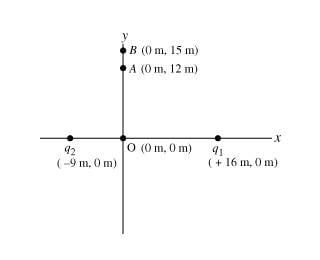# Mastering physics: electric field of point charges

silver421

## Homework Statement

Two point charges are placed on the x axis. The first charge, q1= 8.00 , is placed a distance 16.0m from the origin along the positive x axis; the second charge, q2= 6.00 , is placed a distance 9.00m from the origin along the negative x axis.Calculate the electric field at point A, located at coordinates (0m , 12.0 m).
Give the x and y components of the electric field as an ordered pair. Express your answer in newtons per coulomb to three significant figures.

EAx, EAy=________________________ N/C

## The Attempt at a Solution

thebigstar25
can you please show your attempt of solution so we can help!

silver421
i don't know which equations to use

thebigstar25
E = kq/r^2
Where E is the electric field, k is a constant = 9x10^9, r is the distance from the charge to the point where you want to find the electric field..

Find the electric field produced from q1 at point A , then find the electric field from q2 at point A .. Add them together to get the total electric field at that point ..

Note: the electric field is a vector, when you add you should use vector addition..

I hope it is clear enough for you start solving the question..

tua39657
For these types of problems could someone please explain how you find which of the components x or y cancel. Also how can you tell if E_1 and E_2 are negative or positive? I am pretty sure i found E_1 and E_2 i just don't understand how you can tell to add both together or subtract one from the other.